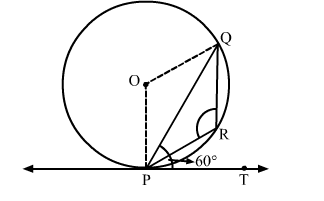# In the given figure, PQ is a chord of a circle with centre O and PT is a tangent.`
Question:

In the given figure, PQ is a chord of a circle with centre O and PT is a tangent. If ∠QPT = 60 , find ∠PRQSolution:

We know that the radius and tangent are perperpendular at their point of contact.
∴∠OPT = 90
Now, ∠OPQ = ∠OPT − ∠QPT = 90 − 60 = 30
Since, OP = OQ as both are radius
∴∠OPQ = ∠OQP = 30          (Angles opposite to equal sides are equal)
Now, In isosceles △POQ
∠POQ + ∠OPQ + ∠OQP = 180       (Angle sum property of a triangle)
⇒ ∠POQ = 180 − 30 − 30 = 120
Now, ∠POQ + reflex ∠POQ = 360         (Complete angle)
⇒ reflex ∠POQ = 360 − 120 = 240
We know that the angle subtended by an arc at the centre is double the angle subtended by the arc at any point on the remaining part of the circle.

$\therefore \angle \mathrm{PRQ}=\frac{1}{2}(\operatorname{reflex} \angle \mathrm{POQ})=120^{\circ}$